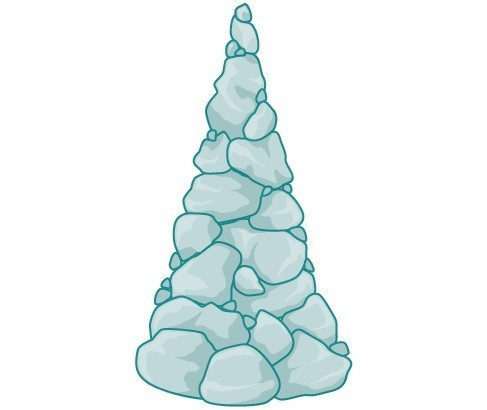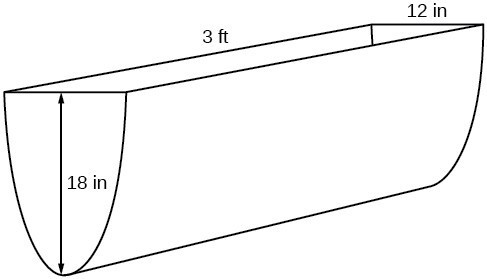### Learning Outcomes

• Find the inverse of a polynomial function.
• Restrict the domain to find the inverse of a polynomial function.

A mound of gravel is in the shape of a cone with the height equal to twice the radius.The volume is found using a formula from geometry.

\begin{align}V&=\frac{1}{3}\pi {r}^{2}h \\[1mm] &=\frac{1}{3}\pi {r}^{2}\left(2r\right) \\[1mm] &=\frac{2}{3}\pi {r}^{3} \end{align}

We have written the volume $V$ in terms of the radius $r$. However, in some cases, we may start out with the volume and want to find the radius. For example: A customer purchases 100 cubic feet of gravel to construct a cone shape mound with a height twice the radius. What are the radius and height of the new cone? To answer this question, we use the formula

$r=\sqrt{\dfrac{3V}{2\pi }}$

This function is the inverse of the formula for $V$ in terms of $r$.

In this section, we will explore the inverses of polynomial and rational functions and in particular the radical functions we encounter in the process.

## Radicals as Inverse Polynomial Functions

Recall that two functions $f$ and $g$ are inverse functions if for every coordinate pair in $f$, $(a, b)$, there exists a corresponding coordinate pair in the inverse function, $g$, $(b, a)$. In other words, the coordinate pairs of the inverse functions have the input and output interchanged.

For a function to have an inverse function the function to create a new function that is one-to-one and would have an inverse function.

For example suppose a water runoff collector is built in the shape of a parabolic trough as shown below. We can use the information in the figure to find the surface area of the water in the trough as a function of the depth of the water.Because it will be helpful to have an equation for the parabolic cross-sectional shape, we will impose a coordinate system at the cross section, with $x$ measured horizontally and $y$ measured vertically, with the origin at the vertex of the parabola.From this we find an equation for the parabolic shape. We placed the origin at the vertex of the parabola, so we know the equation will have form $y\left(x\right)=a{x}^{2}$. Our equation will need to pass through the point (6, 18), from which we can solve for the stretch factor $a$.

\begin{align} 18&=a{6}^{2} \\[1mm] a&=\frac{18}{36} \\[1mm] a&=\frac{1}{2} \end{align}

Our parabolic cross section has the equation

$y\left(x\right)=\frac{1}{2}{x}^{2}$

We are interested in the surface area of the water, so we must determine the width at the top of the water as a function of the water depth. For any depth $y$ the width will be given by $2x$, so we need to solve the equation above for $x$ and find the inverse function. However, notice that the original function is not one-to-one, and indeed, given any output there are two inputs that produce the same output, one positive and one negative.

To find an inverse, we can restrict our original function to a limited domain on which it is one-to-one. In this case, it makes sense to restrict ourselves to positive $x$ values. On this domain, we can find an inverse by solving for the input variable:

\begin{align}y&=\frac{1}{2}{x}^{2} \\[1mm] 2y&={x}^{2} \\[1mm] x&=\pm \sqrt{2y} \end{align}

This is not a function as written. We are limiting ourselves to positive $x$ values, so we eliminate the negative solution, giving us the inverse function we’re looking for.

$y=\dfrac{{x}^{2}}{2},\text{ }x>0$

Because $x$ is the distance from the center of the parabola to either side, the entire width of the water at the top will be $2x$. The trough is 3 feet (36 inches) long, so the surface area will then be:

\begin{align}\text{Area}&=l\cdot w \\[1mm] &=36\cdot 2x \\[1mm] &=72x \\[1mm] &=72\sqrt{2y} \end{align}

This example illustrates two important points:

1. When finding the inverse of a quadratic, we have to limit ourselves to a domain on which the function is one-to-one.
2. The inverse of a quadratic function is a square root function. Both are toolkit functions and different types of power functions.

Functions involving roots are often called radical functions. While it is not possible to find an inverse of most polynomial functions, some basic polynomials do have inverses. Such functions are called invertible functions, and we use the notation ${f}^{-1}\left(x\right)$.

Warning: ${f}^{-1}\left(x\right)$ is not the same as the reciprocal of the function $f\left(x\right)$. This use of –1 is reserved to denote inverse functions. To denote the reciprocal of a function $f\left(x\right)$, we would need to write ${\left(f\left(x\right)\right)}^{-1}=\frac{1}{f\left(x\right)}$.

An important relationship between inverse functions is that they “undo” each other. If ${f}^{-1}$ is the inverse of a function $f$, then $f$ is the inverse of the function ${f}^{-1}$. In other words, whatever the function $f$ does to $x$, ${f}^{-1}$ undoes it—and vice-versa. More formally, we write

${f}^{-1}\left(f\left(x\right)\right)=x,\text{for all }x\text{ in the domain of }f$

and

$f\left({f}^{-1}\left(x\right)\right)=x,\text{for all }x\text{ in the domain of }{f}^{-1}$

### A General Note: Verifying Two Functions Are Inverses of One Another

Two functions, $f$ and $g$, are inverses of one another if for all $x$ in the domain of $f$ and $g$.

$g\left(f\left(x\right)\right)=f\left(g\left(x\right)\right)=x$

### How To: Given a polynomial function, find the inverse of the function by restricting the domain in such a way that the new function is one-to-one.

1. Verify that $f$ is a one-to-one function.
2. Replace $f\left(x\right)$ with $y$.
3. Interchange $x$ and $y$.
4. Solve for $y$, and rename the function ${f}^{-1}\left(x\right)$.

### Example: Verifying Inverse Functions

Show that $f\left(x\right)=\dfrac{1}{x+1}$ and ${f}^{-1}\left(x\right)=\dfrac{1}{x}-1$ are inverses, for $x\ne 0,-1$ .

### Try It

Show that $f\left(x\right)=\dfrac{x+5}{3}$ and ${f}^{-1}\left(x\right)=3x - 5$ are inverses.

### Example: Finding the Inverse of a Cubic Function

Find the inverse of the function $f\left(x\right)=5{x}^{3}+1$.

### Try It

Find the inverse function of $f\left(x\right)=\sqrt{x+4}$.

So far we have been able to find the inverse functions of cubic functions without having to restrict their domains. However, as we know, not all cubic polynomials are one-to-one. Some functions that are not one-to-one may have their domain restricted so that they are one-to-one, but only over that domain. The function over the restricted domain would then have an inverse function. Since quadratic functions are not one-to-one, we must restrict their domain in order to find their inverses.

### A General Note: Restricting the Domain

If a function is not one-to-one, it cannot have an inverse function. If we restrict the domain of the function so that it becomes one-to-one, thus creating a new function, this new function will have an inverse function.

### How To: Given a polynomial function, restrict the domain of a function that is not one-to-one and then find the inverse.

1. Restrict the domain by determining a domain on which the original function is one-to-one.
2. Replace $f(x)$ with $y$.
3. Interchange $x$ and $y$.
4. Solve for $y$, and rename the function or pair of function ${f}^{-1}\left(x\right)$.
5. Revise the formula for ${f}^{-1}\left(x\right)$ by ensuring that the outputs of the inverse function correspond to the restricted domain of the original function.

### Example: Restricting the Domain to Find the Inverse of a Polynomial Function

Find the inverse function of $f$:

1. $f\left(x\right)={\left(x - 4\right)}^{2}, x\ge 4$
2. $f\left(x\right)={\left(x - 4\right)}^{2}, x\le 4$

### Example: Finding the Inverse of a Quadratic Function When the Restriction Is Not Specified

Restrict the domain and then find the inverse of

$f\left(x\right)={\left(x - 2\right)}^{2}-3$.

### Try It

Find the inverse of the function $f\left(x\right)={x}^{2}+1$, on the domain $x\ge 0$.

Watch the following video to see more examples of how to restrict the domain of a quadratic function to find it’s inverse.

## Solving Applications of Radical Functions

Notice that the functions from previous examples were all polynomials, and their inverses were radical functions. If we want to find the inverse of a radical function, we will need to restrict the domain of the answer because the range of the original function is limited.

### How To: Given a radical function, find the inverse.

1. Determine the range of the original function.
2. Replace $f(x)$ with $y$, then solve for $x$.
3. If necessary, restrict the domain of the inverse function to the range of the original function.

### Example: Finding the Inverse of a Radical Function

Restrict the domain and then find the inverse of the function $f\left(x\right)=\sqrt{x - 4}$.

### Try It

Restrict the domain and then find the inverse of the function $f\left(x\right)=\sqrt{2x+3}$.

### Solving Applications of Radical Functions

Radical functions are common in physical models, as we saw in the section opener. We now have enough tools to be able to solve the problem posed at the start of the section.

### Example: Solving an Application with a Cubic Function

A mound of gravel is in the shape of a cone with the height equal to twice the radius. The volume of the cone in terms of the radius is given by

$V=\frac{2}{3}\pi {r}^{3}$

Find the inverse of the function $V=\frac{2}{3}\pi {r}^{3}$ that determines the volume $V$ of a cone and is a function of the radius $r$. Then use the inverse function to calculate the radius of such a mound of gravel measuring 100 cubic feet. Use $\pi =3.14$.

## Determining the Domain of a Radical Function Composed with Other Functions

When radical functions are composed with other functions, determining domain can become more complicated.

### Example: Finding the Domain of a Radical Function Composed with a Rational Function

Find the domain of the function $f\left(x\right)=\sqrt{\dfrac{\left(x+2\right)\left(x - 3\right)}{\left(x - 1\right)}}$.

## Finding Inverses of Ratinal Functions

As with finding inverses of quadratic functions, it is sometimes desirable to find the inverse of a rational function, particularly of rational functions that are the ratio of linear functions, such as in concentration applications.

### Example: Finding the Inverse of a Rational Function

The function $C=\dfrac{20+0.4n}{100+n}$ represents the concentration $C$ of an acid solution after $n$ mL of 40% solution has been added to 100 mL of a 20% solution. First, find the inverse of the function; that is, find an expression for $n$ in terms of $C$. Then use your result to determine how much of the 40% solution should be added so that the final mixture is a 35% solution.

### Try It

Find the inverse of the function $f\left(x\right)=\dfrac{x+3}{x - 2}$.

Watch this video to see another worked example of how to find the inverse of a rational function.

## Key Concepts

• The inverse of a quadratic function is a square root function.
• If ${f}^{-1}$ is the inverse of a function $f$, then $f$ is the inverse of the function ${f}^{-1}$.
• While it is not possible to find an inverse of most polynomial functions, some basic polynomials are invertible.
• To find the inverse of certain functions, we must restrict the function to a domain on which it will be one-to-one.
• When finding the inverse of a radical function, we need a restriction on the domain of the answer.
• Inverse and radical and functions can be used to solve application problems.

## Glossary

invertible function
any function that has an inverse function